# How to Calculate the Interquartile Range in Excel?

• Last Updated : 28 Jul, 2021

In statistics, the five-number summary is mostly used as it gives a rough idea about the dataset. It is basically a summary of the dataset describing some key features in statistics. The five key features are :

1. Minimum value: It is the minimum value in the data set.
2. First Quartile, Q1: It is also known as the lower quartile where 25% of the scores fall below it.
3. Median (middle value) or second quartile: It is basically the mid-value in the dataset.
4. Third Quartile, Q3: It is also known as the Upper quartile in which 25% of the data is above it and the rest 75% falls below it.
5. Maximum value: It is the maximum value in the dataset.

Using two quartiles of the five-number summary we can easily calculate the IQR abbreviated as Interquartile Range.

In this article, we are going to see how to calculate the Interquartile range in Excel using a sample dataset as an example.

## Interquartile Range

In terms of Mathematics, it is basically defined as the difference between the third quartile (75th  percentile) and the first quartile (25th percentile).

`Q3-Q1`

IQR denotes the middle 50% hence also known as midspread or H-spread in statistics. It can be easily observed using a box plot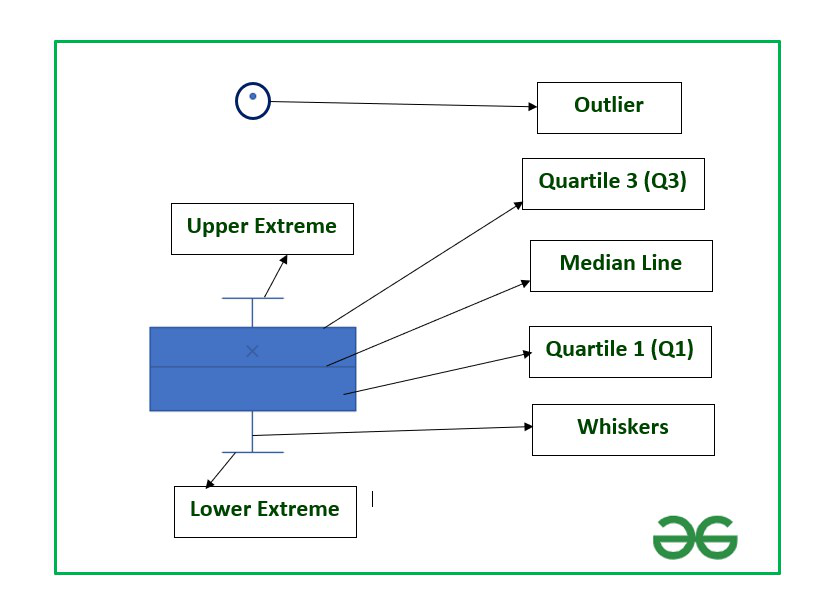The vertical lines of the rectangular box plot denote the Interquartile range which lies between Quartile 1 and Quartile 3.

Example: Consider the dataset consisting of the BMI of ten students in a class.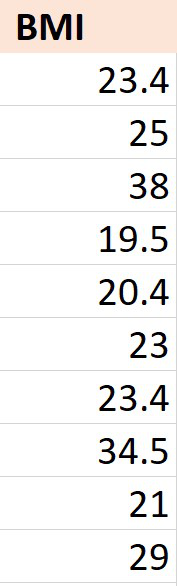Now, in order to calculate the IQR we need to first calculate the two quartile values Q1 and Q3. The function used is :

```QUARTILE(Array,quart)         // Used in Excel 2007 version and lower
or
QUARTILE.INC(Array,quart)    // Used in latest version of Excel

Array : Cell range
quart : The five quart values from 0 to 4

0- Minimum value
1- First Quartile (25 percent)
2- Median Value (50 percentile)
3- Third Quartile (75 percentile)
4- Maximum value```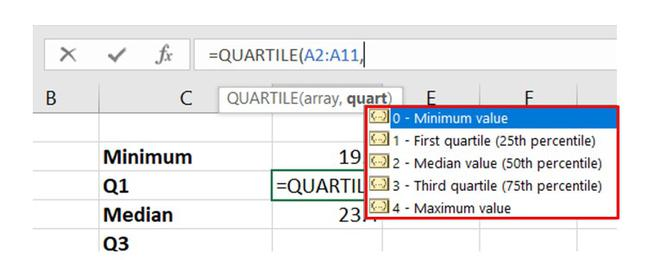quart values

### Calculation

Follow the below steps to calculate the same:

Step 1: Insert the dataset.

Step 2: Select any cell where you want to write the formula to calculate the values of Q1, Q3, and IQR.

Step 3: First find the values of Q1 and Q3 using the quart values as 1 and 3 respectively.

The dataset is stored in column “A” of the worksheet and the observations are stored from cell A2 to A11.

So the array will start from A2 and end at A11.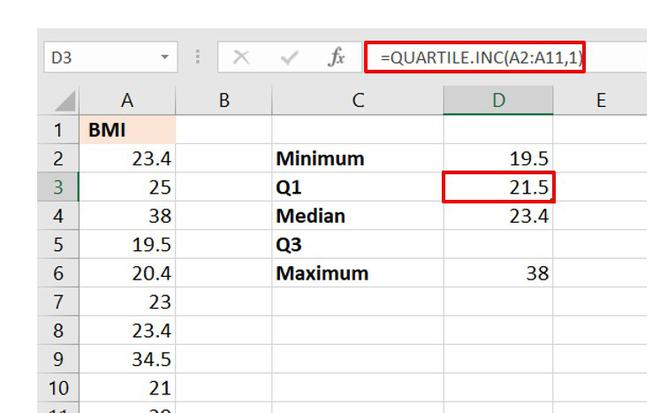Calculation of Q1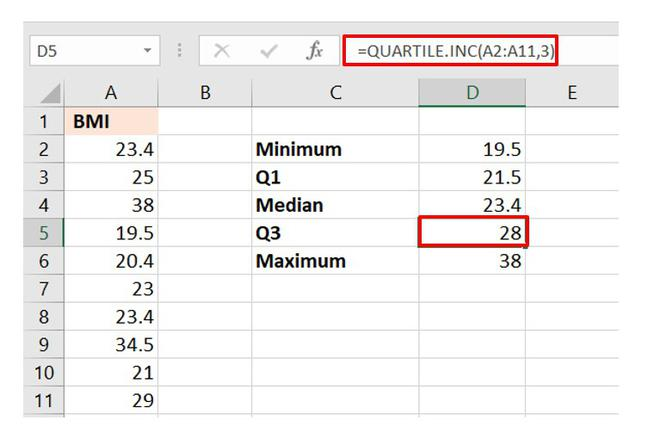Calculation of Q3

You can also find the remaining three parameters of a five-number summary using the same formula just by changing the quart value. But to find the IQR, we only need the values of Q1 and Q3.

The value of Q3 is stored in cell D4 and that of Q1 in cell D3. The formula will be :

`=Cell_no_Q3-Cell_no_Q1`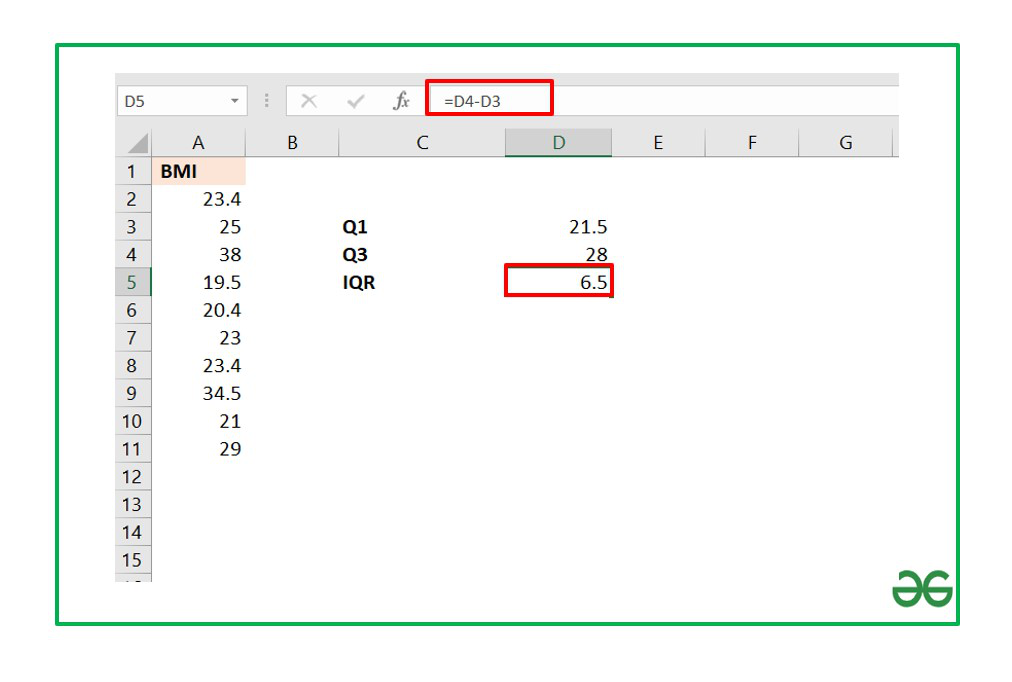The Interquartile range for the above dataset turns out to be 6.5.

My Personal Notes arrow_drop_up